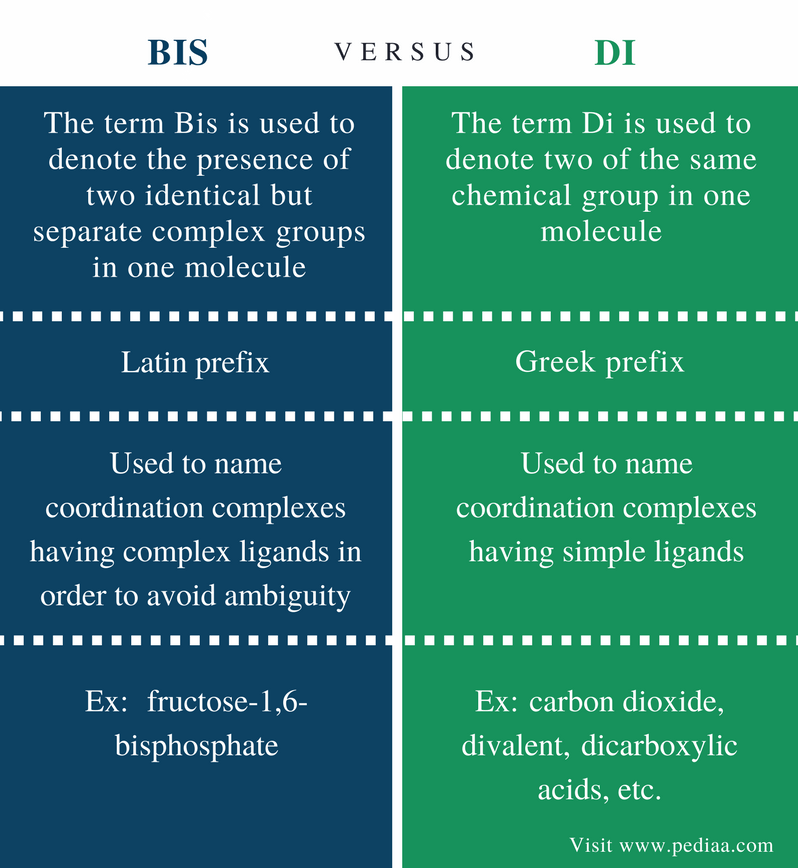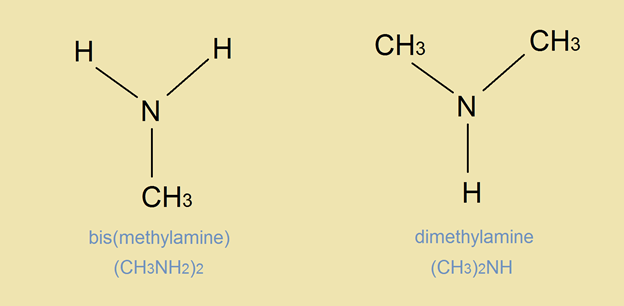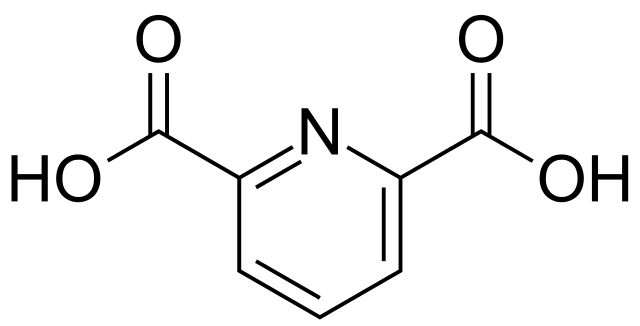# Difference Between Bis and Di

## Main Difference – Bis vs Di

The terms Bis and Di are prefixes that are used in naming chemical compounds. The term Bis is used to denote the presence of two identical but separated complex groups in one molecule. For example, in fructose-1,6-bisphosphate, fructose sugar molecule is phosphorylated at 1 and 6 carbon atoms. However, it is a Latin prefix. On the other hand, the term Di is used to say “two” or “twice”. It is a Greek prefix. Basically, prefixes Bis and Di are the same in their meaning. They are different from each other in the origin and usage. The main difference between Bis and Di is that Bis is used to denote the presence of two identical but separate complex groups in one molecule whereas Di is used to denote two of the same chemical group in one molecule.

### Key Areas Covered

1. What is Bis
– Definition, Usage, Examples
2. What is Di
– Definition, Usage, Examples
3. What are the Similarities Between Bis and Di
– Outline of Common Features
4. What is the Difference Between Bis and Di
– Comparison of Key Differences

Key Terms: Atomicity, Bis, Di, Prefix, Valency## What is Bis

The term Bis is used to denote the presence of two identical but separated complex groups in one molecule. Basically, it means “two” or “twice”. Bis is a Latin prefix. The term “Di” also express the same meaning, but their applications in coordination chemistry is different. The term bis is used to name coordination complexes having complex ligands in order to avoid ambiguity. The term Di is used to name coordination complexes having simple ligands.Figure 1: The term bis is used instead of di in order to avoid any confusion in coordination chemistry.

This is because complex ligands have multiple prefixes with the ligand name. When naming the whole compound, the prefix used to name the number of ligands and the prefixes used in naming one ligand may be confusing due to complexity. Therefore, the term “bis” is used there instead of saying “di”.

## What is Di

The term Di is used to say “two” or “twice”. It is a prefix used to name chemical compounds having two of the same chemical group in one molecule. Give below are some examples.

• Carbon dioxide (CO2) – two oxygen atoms are bonded to the same carbon atoms. Therefore “dioxide” means “two oxygen atoms”.
• Dichloramine (NHCl2) two chlorine atoms are bonded to the nitrogen atom.
• Water molecule is named dihydrogen oxide since its chemical formula is H2O, and there are two hydrogen atoms bonded to the oxygen atom.
• H2S is named as dihydrogen sulfide since it has two hydrogen atoms bonded to a sulfur atom.

The term Di is also used to give the valency of an atom. For example, magnesium is a divalent atom whose valency is two. Therefore, magnesium can form Mg+2 cation. This cation is then named as divalent cation whose valency is two.

In organic chemistry, the prefix di is used to name organic molecules having two functional groups. Followings are some examples.

• A dicarboxylic acid is a carboxylic acid containing two carboxyl functional groups (-COOH). Ex: oxalic acid is the simplest dicarboxylic acid.
• A diamine is an amine consists of two amine groups (-NH2). Ex: ethylene diamine.
• A diol is an alcohol composed of two hydroxyl groups (-OH). Ex: Ethylene glycol.Figure 2: A Dicarboxylic Acid

In general, the prefix di is used to give the atomicity of a compound. For example, HCl (hydrogen chloride) is a diatomic molecule having two atoms.

When naming coordination compounds, we use the prefix di to give the number of ligands present in the complex. Ex: Dioxalato cobalt (III) is [Co(C2O4)2]. There are two oxalate ligands bonded to the cobalt ion. However, the term Di is used when simple ligands are present. Otherwise, the term “bis” is used.

## Similarity Between Bis and Di

• Both prefixes Bis and Di basically express the same meaning.

## Difference Between Bis and Di

### Definition

Bis: The term Bis is used to denote the presence of two identical but separated complex groups in one molecule.

Di: The term Di means “two” or “twice” and is used to denote two of the same chemical group in one molecule.

### Origin

Bis: Bis is a Latin prefix.

Di: DI is a Greek prefix.

### Application in Coordination Chemistry

Bis: The term bis is used to name coordination complexes having complex ligands in order to avoid ambiguity.

Di: The term di is used to name coordination complexes having simple ligands.

### Examples

Bis: Some examples for naming compounds using Bis include fructose-1,6-bisphosphate,

Di: Some examples include inorganic compounds such as CO2 (carbon dioxide), valency such as valency of Magnesium (divalent), organic compounds containing two functional groups such as dicarboxylic acids, etc.

### Conclusion

The terms bis and di basically have the same meaning. But they are different from each other in their usage and the origin. The main difference between Bis and Di is that Bis is used to denote the presence of two identical but separated complex groups in one molecule whereas Di is used to denote two of the same chemical group in one molecule.

##### Reference:

1. “BIS.” Wikipedia, Wikimedia Foundation, 25 Dec. 2017, Available here.
2. “When are the prefixes bis, tris etc. used in nomenclature of complexs?” Inorganic chemistry – Chemistry Stack Exchange, Available here.

##### Image Courtesy:

1. “Pyridine-2,6-dicarboxylic acid 200” By Emeldir (talk) – Own work (Public Domain) via Commons Wikimedia